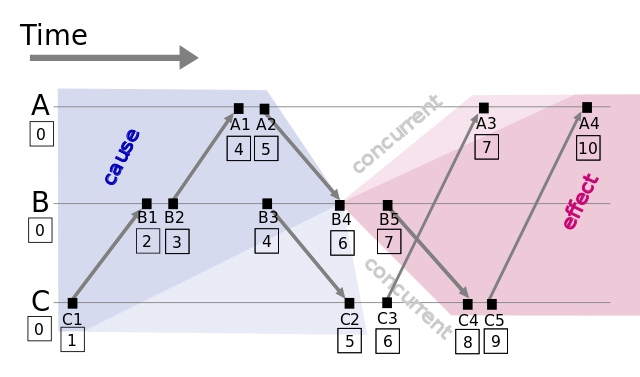# Lamport逻辑时钟（Larmport Logical Clock）0

## 什么是逻辑时钟

• 逻辑时钟是为了区分现实中物理时钟提出来的概念。
• 一般情况下我们提到的时间都是指物理时间，但实际上很多应用中，只要所有机器有相同的时间就够了，这个时间不一定要跟实际时间相同。更进一步，如果两个节点之间不进行交互，那么它们的时间甚至都不需要同步。
• 因此问题的关键点在于节点间的交互要在事件的发生顺序上达成一致，而不是对于时间达成一致。

## 如何实现逻辑时钟

### Lamport逻辑时钟

Clock Condition. 对于任意事件$a$, $b$：如果$a \rightarrow b$（$\rightarrow$表示$a$先于$b$发生），那么$𝐶(a)<𝐶(b)$；反之不然, 因为有可能是并发事件。
C1. 如果$a$和$b$都是进程$P_i$里的事件，并且$a$在$b$之前，那么$C_i(a)<C_i(b)$;
C2. 如果$a$是进程$P_i$里关于某消息的发送事件，$b$是另一进程$P_j$里关于该消息的接收事件，那么$C_i(a)<C_j(b)$;

Lamport逻辑时钟原理如下：1. 每个事件对应一个Lamport时间戳，初始值为0
2. 如果事件在节点内发生，本地进程中的时间戳加1
3. 如果事件属于发送事件，本地进程中的时间戳加1并在消息中带上该时间戳
4. 如果事件属于接收事件，本地进程中的时间戳 = Max(本地时间戳，消息中的时间戳) + 1

$C1 \rightarrow B1 \rightarrow B2 \rightarrow A1 \rightarrow B3 \rightarrow A2 \rightarrow C2 \rightarrow B4 \rightarrow C3 \rightarrow A3 \rightarrow B5 \rightarrow C4 \rightarrow C5 \rightarrow A4$

1. http://research.microsoft.com/users/lamport/pubs/time-clocks.pdf
2. https://en.wikipedia.org/w/index.php?title=Network_Time_Protocol&oldid=973055798
0. https://blog.xiaohansong.com/lamport-logic-clock.html “分布式系统：Lamport 逻辑时钟”